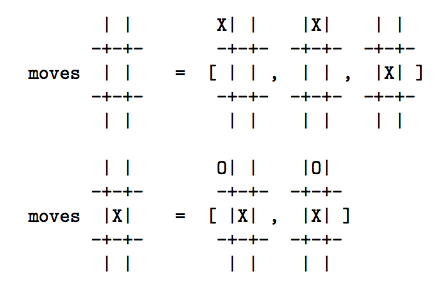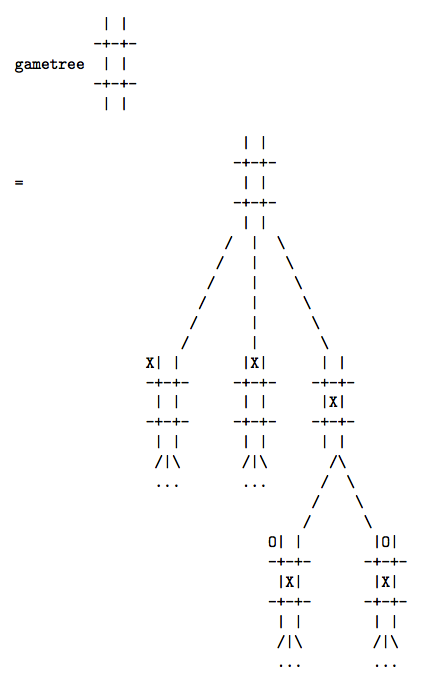Why Functional Programming Matters 函數式程序設計爲什麼至關重要

## 2.與結構化程序設計的相似性

“不使用goto”等等這一類特性，對於這一提升沒什麼作用。這些特性促進了“程序設計的小改良”，然而模塊化設計卻促進了“程序設計的大進化”。因此，程序員在FORTRAN或彙編語言中都可以享受結構化程序設計帶來的好處，哪怕那需要一點額外的工作。

## 3.把函數粘起來

``````listof X :: = nil | cons X (listof X)
``````

``````[] 表示 nil
 表示 cons 1 nil
[1 2 3] 表示 cons 1 ( cons 2 ( cons 3 nil ))
``````

``````sum nil = 0
``````

``````sum (cons num list) = num + sum list
``````

sum nil = 0

sum (cons num list) = num + sum list

``````sum = reduce add 0
``````

``````add x y = x + y
``````

``````(reduce f x) nil = x
(reduce f x)(cons num list) = f num ((reduce f x) list)
``````

``````product = reduce multiply 1
``````

``````anytrue = reduce or false
``````

``````alltrue = reduce and true
``````

``````cons 1 (cons 2 (cons 3 nil))
``````

``````add 1 (add 2 (add 3 0)) = 6
``````

`(reduce multiply 1)`將其轉換爲：

``````multiply 1 (mulitiply 2 (mulitiply 3 1)) = 6
``````

``````append a b = reduce cons b a
``````

``````append [1,2] [3,4] = reduce cons [3,4] [1,2]
= (reduce cons [3,4]) (cons 1 ( cons 2 nil ))
= cons 1 ( cons 2 [3,4]))
= [1,2,3,4] （將cons替換爲cons，將nil替換爲[3,4]）
``````

``````doubleall = reduce doubleandcons nil
doubleandcons num list = cons ( 2*num ) list
``````

`doubleandcons`可以進一步模塊化，首先分解爲：

``````doubleandcons = fandcons double
double n = 2*n
fandcons f elem list = cons (f elem) list
``````

``````fandcons f = cons . f
``````

``````(f . g) h = f(g h)
``````

``````fandcons f elem
= (cons . f) elem
= cons (f elem)
``````

``````fandcons f elem list = cons (f elem) list
``````

``````doubleall = reduce (cons . double) nil
``````

``````doubleall = map double
map f = reduce (cons . f) nil
``````

``````summatrix = sum . map sum
``````

`map sum`使用函數`sum`分別計算所有行的元素之和，而後最左邊的`sum`將每一行的元素之和累加起來，從而得到整個矩陣的累加和。

``````treeof X ::= node X (listof (treeof X))
``````

``````           1 o
/\
/  \
/    \
2 o     o 3
|
|
|
o 4
``````

``````node 1(cons
(node 2 nil)
(cons (node 3
(cons (node 4 nil) nil)
)
nil))
``````

``````redtree f g a (node label subtrees) = f label (redtree' f g a subtrees )
redtree' f g a (cons subtree rest) = g (redtree f g a subtree) (redtree' f g a rest)
redtree' f g a nil = a
``````

［這相當於`f`取代`node``g`取代`cons``a`取代`nil`。］

``````sumtree = redtree add add 0
``````

``````add 1
0))
= 10
``````

``````labels = redtree cons append nil
``````

``````cons 1
(append (cons 2 nil)
(append (cons 3
(append (cons 4 nil) nil))
nil))
= [1,2,3,4]
``````

``````maptree f = redtree (node . f) cons nil
``````

## 4.把程序粘起來

``````g (f input)
``````

## 4.1.牛頓－拉夫森求根法

``````a(n+1) = (a(n) + N/a(n)) / 2
``````

``````a = (a + N/a) / 2
2a = a + N/a
a = N/a
a*a = N
a = squareroot(N)
``````

``````C N IS CALLED ZN HERE SO THAT IT HAS THE RIGHT TYPE
X = A0
Y = A0 + 2.*EPS
C THE VALUE OF Y DOES NOT MATTER SO LONG AS ABS(X-Y).GT.EPS
100 IF (ABS(X-Y).LE.EPS) GOTO 200
Y = X
X = (X + ZN/X) / 2
200 CONTINUE
C THE SQUARE ROOT OF ZN IS NOW IN X
``````

［這是一段FORTRAN的程序，C代表註釋行。.LE.是“Less than or Equal to”（小於或等於）的縮寫，同理.GT.是“大於”的意思。]

``````next N x = (x + N/x) / 2
``````

``````[a0, f a0, f(f a0), f(f(f a0)), ...]
``````

``````repeat f a = cons a (repeat f (f a))
``````

``````repeat (next N) a0
``````

`repeat`是一個具有“無窮”輸出的函數的例子——但這沒關係，因爲超出程序其餘部分需求的近似值並不會被計算。無窮性只是潛在的：它只說明，只要有需求，就可以計算出任意數量的近似值，`repeat`本身不會強加任何限制。

``````within eps (cons a (cons b rest)) = {
= b, 如果 abs(a-b) <= eps
= within eps (cons b rest), 其他情況
}
``````

``````sqrt a0 eps N = within eps (repeat (next N) a0)
``````

``````relative eps (cons a (cons b rest)) =
= b, 如果 abs(a-b) <= eps*abs b
= relative eps (cons b rest), 其他情況
``````

［注意：`relative`裏的`eps``within`裏的`eps`定義是不同的！前者是絕對誤差後者是相對誤差！］

## 4.2.數值微分

``````easydiff f x h = (f(x+h)-f x) / h
``````

``````differentiate h0 f x = map (easydiff f x) (repeat halve h0)
halve x = x/2
``````

``````within eps (differentiate h0 f x)
``````

``````微分的精確值 ＋ 一個關於h的誤差項
``````

``````a(i)   = A + B*(2**n)*(h**n)
a(i+1) = A + B*(h**n)
``````

``````A=(a(i+1)*(2**n) - a(i))/(2**n - 1)
``````

``````elimerror n (cons a (cons b rest)) =
= cons ((b*(2**n)-a)/(2**n-1)) (elimerror n (cons b rest))
``````

``````order (cons a (cons b (cons c rest))) =
= round(log2( (a-c)/(b-c) - 1 ))
round x = 最接近x的整數
log2 x  = x以2爲底的對數
``````

``````improve s = elimerror (order s) s
``````

``````within eps (improve (differentiate h0 f x))
``````

`improve`只對利用一個不斷減半的參數`h`計算得到的近似值序列適用。但是，如果`improve`作用於這樣的序列，那麼其結果也是一個這樣的序列！這意味着一個近似值序列可以優化不止一次。每一次優化的過程中，都有一個不同的誤差項被消除，因此優化產生的序列收斂得越來越快。因此，可以非常高效地計算導數：

``````within eps (improve (improve (improve (differentiate h0 f x))
``````

``````super s = map second (repeat improve s)
second (cons a (cons b rest)) = b
``````

`super`函數使用`repeat improve`來生成一個不斷被優化的近似值的序列的序列。［就是說，生成一個序列，其中每一個元素是一個近似值序列，而這個元素是用前一個元素優化得到的。］同時，`super`提取出每個近似值序列中的第二個元素，構造出一個新的序列（已經確認，第二元素是最佳選擇——它比首元更精確，而且不需要額外的計算）。這個算法的確非常複雜——更多的近似值被計算的同時，它使用了不斷優化的數值方法。可以用下面的程序非常非常高效地計算導數：

``````within eps (super (differentiate h0 f x))
``````

## 4.3.數值積分

``````easyintegrate f a b = (f a + f b)*(b-a)/2
``````

``````intergrate f a b = cons (easyintergrate f a b)
(map addpair (zip (intergrate f a mid)
(intergrate f mid b)))

``````

`zip`是另一個標準的表處理函數。它讀取兩個列表，並返回一個有序對的列表，每個有序對由兩個輸入列表中對應的元素組成。從而第一對由列表一和列表二的首元組成，第二對由列表一和列表二的第二個元素組成，以此類推。`zip`可以定義爲：

``````zip (cons a s) (cons b t) = cons (pair a b) (zip s t)
``````

``````intergrate f a b = interg f a b (f a) (f b)
integ f a b fa fb = cons ((fa+fb)*(b-a)/2)
(map addpair (zip (interg f a m fa fm)
(interg f m b fm fb)))

fm = f m
``````

`integrate`給出了一個不斷趨近準確值的積分近似值列表，正如`differentiate`在上一小節中所做的一樣。因此可以寫出計算式以求出所需任意精度的積分值，如下：

``````within eps (intergrate f a b)
relative eps (integrate f a b)
``````

``````super (intergrate sin 0 4)
improve (intergrate f 0 1)

``````

（後一個序列是用於計算`pi/4`的“第八階方法”。其中的第二個近似值只需要計算5次`f`的取值，但卻具有5位準確數字。）

## 5.人工智能中的例子

``````moves: position -> listof position
````````````reptree f a = node a (map (reptree f) (f a))
``````

``````gametree p = reptree moves p
``````alpha-beta算法從一個給定的局勢出發，就遊戲的發展將會是有利還是不利作出判斷。然而，要做到這一點，它必須能夠在不考慮下一步的情況下粗略地估計某一個局勢的“價值”。在後繼局勢不可預測時必須使用這一函數，它也可以用來對算法進行先期引導。靜態估價的結果是從計算機的角度考慮的，是對該局勢的前途的度量（假設在遊戲中計算機與人對抗）。結果越大，局勢對計算機而言越好。結果越小，局勢越糟。最簡單的此類函數將會，比如說，對計算機確定勝利的局勢返回+1，對計算機確定失敗的局勢返回-1，而對其它的局勢返回0。在現實中，靜態估價函數會衡量各種使局勢“看上去不錯”的因素。例如，具體的好處，以及象棋中對中心的控制。假定有這樣一個函數：

``````static: position -> number
``````

［所謂“真值”（true value），可能是我翻譯得不好，此處理解爲類似“真正的價值”的意思吧，是一個量度，不是邏輯學裏的0和1哦。］

``````maximise (node n sub) = max (map minimise sub)
minimise (node n sub) = min (map maximise sub)
``````

``````maximise (node n nil) = n
maximise (node n sub) = max (map minimise sub)
maximise (node n nil) = n
maximise (node n sub) = min (map minimise sub)
``````

``````evaluate = maximise . maptree static . gametree
``````

``````prune 0 (node a x) = node a nil
prune n (node a x) = node a (map (prune (n-1)) x)
``````

`(prune n)`取一棵樹作爲參數並“剪去”與根結點的距離超過n的所有結點。如果一棵博弈樹被剪枝，那麼將強制`maximise`對深度爲`n`的結點執行靜態估價而不是進一步遞歸。因此`evaluate`可以被定義爲：

``````evaluate = maximise . maptree static . prune 5 . gametree
``````

``````prune 5 . gametree
``````

``````maptree static . prune 5 . gametree
``````

``````          max
/ \
/   \
/     \
/       \
min       min
/ \       / \
/   \     /   \
1     2   0     ?
``````

``````maximise = max . maximise'
``````

`minimise`可以用類似的方法分解。由於`maximise``minimise`是完全對稱的，故我們將只討論`maximise`，而假定`minimise`也照此處理。）一旦這樣分解之後，`maximise`可以使用`minimise'`來發現`minimise`將對哪些數字求最小值，並且不再使用`minimise`本身。而後便可以在不查看某些數字的情況下便將它們丟棄。由於惰性求值的存在，如果`maxmise`並不會查看所有的數字列表，那麼一部分列表將不會被計算，這是對計算機時間的潛在節約。

`max``maximise`中“約分出來”是很簡單的，得到：

``````maximise' (node n nil) = cons n nil
maximise' (node n l) = map minimise l
= map (min . minimise') l
= map min (map minimise' l)
= mapmin (map minimise' l)

``````

``````mapmin (cons nums rest) =
= cons (min nums) (omit (min nums) rest)
``````

``````omit pot nil = nil
omit pot (cons nums rest) =
= omit pot rest, if minleq nums pot
= cons (min nums) (omit (min nums) rest), otherwise
``````

`minleq`以一個數字列表和一個潛在最大值爲參數，如果列表的最小值小於或等於潛在最大值就返回真。要完成這一工作，它並不需要掃描整個列表！如果列表中有任意一個元素小於或等於潛在最大值，那麼列表的最小值肯定也是如此。該特別元素之後的所有元素都是無關緊要的——它們就像是上面例子中的問號一樣。因此`minleq`可以被定義爲：

``````minleq nil pot = false
minleq (cons num rest) port = true, if numn2
``````

``````evaluate = max . maximise' . highfirst . maptree static . prune 8 . gametree
``````

``````taketree n = redtree (nodett n) cons nil
nodett n label sub = node label (take n sub)
``````

`taketree`將樹上所有的結點替換爲最多有n個子結點的結點，它使用了函數`(take n)`，而該函數返回列表的前n個元素（如果列表比n短，那麼返回的元素就少一些）。

``````prune 0 (node pos sub) = node pos (map (prune 0) sub), if dynamic pos
``````

## 參考文獻

• [AS86] H.Abelson,G.J.Sussman. 計算機程序的構造與解釋. 麻省理工學院出版社,波士頓,1986
• [Hug89] J.Hughes. 函數式程序設計爲什麼至關重要. 計算機月刊,32(2),1989
• [Hug90] John Hughes. 函數式程序設計爲什麼至關重要. D.Turner主編,函數式編程的研究主題. Addison Wesley,1990
• [MTH90] R.Milner,M.Tofte,R.Harper. Standard ML的定義. 麻省理工學院出版社,1990